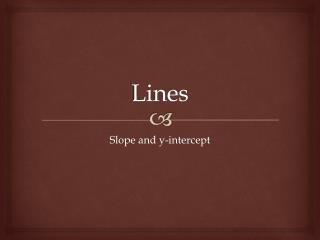DownloadDownload PresentationLines

# Lines

Download Presentation## Lines

- - - - - - - - - - - - - - - - - - - - - - - - - - - E N D - - - - - - - - - - - - - - - - - - - - - - - - - - -
##### Presentation Transcript

1. Lines Slope and y-intercept

2. Lines • Lines have both slope (m) and a y-intercept (b). • Slope describes the steepness of the line as well as its direction. • Positive slope means the lines go up toward the right; negative slope means it goes down to the right. • The larger the slope, the steeper the line. • A slope of 5 is a very steep line, while a slope of 1/5 is very flat. • The y-intercept tells you where the line crosses the y-axis.

3. Slope • Slope is the rise over the run. • We find slope using the equation: • m = • Slope is always a fraction or a whole number. • Slope is telling you how to move to find other points on the lines. I do not know how to move in decimals. • Find the slope of the line that passes through (5,2) and (4,9). • m = • m = • m = - 7

4. Slope • You only get better when you practice, so….. • Find the slope of the line that passes through the points: • 1. (4,4) and (6,6) 2. (3,7) and (4,8) • m = 1 m = 1 • 3. (7,-5) and (-4,-4) 4. (-6,-1) and (7,-6) • m = -1/11 m = -5/13

5. Slope - Intercept • Slope tells you how to move and the y-intercept tells you where the line cross the y-axis. • y = mx + b……you have seen this before • m is the slope and b is the y-intercept. • When giving the y-intercept, give the coordinates as an ordered pair. (0,b) • You can use those two things to graph any line. • y = 2x + 3 • Slope is 2 and the y-intercept is (0,3).

6. Slope-Intercept • Try a couple more……. • 1. y = 5x – 6 2. y = -2x + 3 • 3. y = -1/3x + 6 4. y = 1/2x - 4

7. Slope-Intercept • Now we will practice writing equations. • Remember you have to have a slope (m) and a y-intercept (b) to write an equation. • Write the equation for the line given the slope and intercept. • 1. m = 2 y-intercept (0,5) • 2. m = -8 y-intercept (0,4) • 3. m = ½ y-intercept (0,-3) y = 2x + 5 y = -8x + 4 y = 1/2x - 3

8. Slope-Intercept • Now for the hard ones…… • What happens if you have the slope, but not the y-intercept? • Solve for it!!! • Write the equation of the line that has a slope of 2 and passes through (4,6). • y = mx + b • 6 = 2(4) + b • 6 = 8 + b • b = -2 • y = 2x - 2 Plug in the numbers that you know: m = 2, y = 6, x = 4 Solve for b. Rewrite in slope-intercept form.

9. Slope-Intercept • I know, that was tricky so try it again…. • m = 4 (-1,2) • 2 = 4(-1) + b • 2 = -4 + b • b = 6 • y = 4x + 6 m = -1/2 (4,-6) -6 = -1/2(4) + b -6 = -2 + b b = -4 y = -1/2x - 4